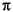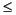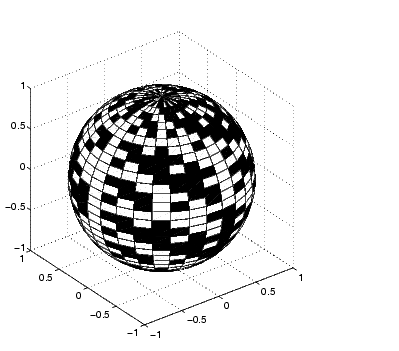3-D VisualizationParametric Surfaces

The functions that draw surfaces can take two additional vector or matrix arguments to describe surfaces with specific x and y data. If `Z` is an m-by-n matrix, `x` is an n-vector, and `y` is an m-vector, then

• ```mesh(x,y,Z,C)
```

describes a mesh surface with vertices having color `C(i,j)` and located at the points

• ```(x(j), y(i), Z(i,j))
```

where `x` corresponds to the columns of `Z` and `y` to its rows.

More generally, if `X,` `Y,` `Z`, and `C` are matrices of the same dimensions, then

• ```mesh(X,Y,Z,C)
```

describes a mesh surface with vertices having color `C(i,j)` and located at the points

• ```(X(i,j), Y(i,j), Z(i,j))
```

This example uses spherical coordinates to draw a sphere and color it with the pattern of pluses and minuses in a Hadamard matrix, an orthogonal matrix used in signal processing coding theory. The vectors `theta` and `phi` are in the range `-``theta`and `-``/2``phi``/2`. Because `theta` is a row vector and `phi` is a column vector, the multiplications that produce the matrices `X`, `Y`, and `Z` are vector outer products.

• ```k = 5;
n = 2^k-1;
theta = pi`*`(-n:2:n)/n;
phi = (pi/2)`*`(-n:2:n)'/n;
X = cos(phi)`*`cos(theta);
Y = cos(phi)`*`sin(theta);
Z = sin(phi)`*`ones(size(theta));
colormap([0 0 0;1 1 1])Surface Plots of Nonuniformly Sampled Data Hidden Line Removal